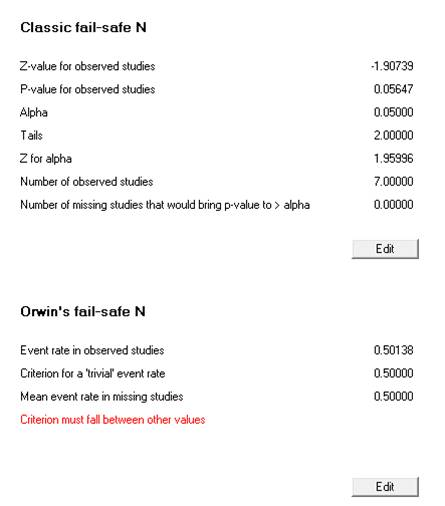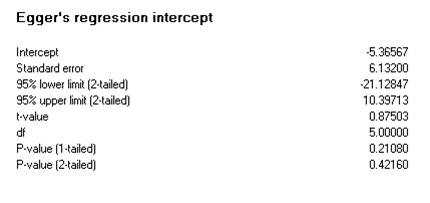Interpretation of results of meta analysis on different types of plot

The previous articles provided an introduction to the results and analysis interface. This module now proceeds towards the interpretation of results. The meta-analysis results involve basic summary statistics, forest plots and model statistics. Besides these, also funnel plot can be obtained to study publication bias amongst the studies in the analysis.

Interpreting the basic summary statistics

Model statistics and forest plot can be viewed by accessing the options as shown in the figures below.

Results for meta analysis

This section shows the results for meta analysis. The yellow band shows the overall summary results at 95% confidence intervalFor the present case study on the association of cholecystitis with gallbladder cancer, the summary effect size is 0.38, with Z-value = -0.69 and p = 0.49. The figure also shows overall summary statistics for both models.

The summary effect size is given as the ‘Point estimate’ with lower and upper limits. The test of null hypothesis uses the Z and p values, to check whether the treatment effect is significant or not. The ‘Heterogeneity’ and ‘Tau-squared’ values give information about the heterogeneity within the studies.

The summary effect size obtained was 0.38 with p-value = 0.49 (>0.05), thus leading to rejection of the alternative hypothesis. This indicates the non significant association of gallstones with the occurrence of gallbladder carcinoma. The I2 statistics for heterogeneity was 97.12 (97.12%), p = 0.00, resulting in acceptance of alternative hypothesis and indicates the presence of ‘significant’ heterogeneity within the studies in taken for review.

Forest plot

The forest plot is the graphical representation of the meta-analysis. The figure below shows the forest plot for dichotomous outcome variable. Here, each row represents individual study results. The effect size measure for present review is ‘Event rate’. The line dividing the graph into two parts is the line of no effect. The boxes represent the individual studies, with their size reflecting the weight of the respective study in the meta-analysis. Bigger the size, more the weight.

The horizontal lines passing through the boxes are the whiskers. The length of whiskers represents the length of the confidence interval. More the length, wider the confidence interval and lower the precision of study results. The diamond shaped object represents the overall summary results. The middle portion represents the overall effect estimate, whereas the width represents the overall confidence interval. If the diamond crosses the line of no effect then the results are not statistically significant. The outcome variables do not have a standard graphical orientation and thus the software allows to name the axis.

Thus, in the shown forest plot, the region to the left of the line of no effect represents no association. Whereas, the region on right represents the association. Name the axis labels, as per their study design, as shown below.

Funnel plot

The funnel plot refers to a scatter plot presenting the estimates of effect sizes of individual studies. It is a plot of estimates against the standard error, which gives a measure of the precision of the effect size. The trials having small sample size gives less precise estimates, due to the large size of the standard error. Whereas, trial having large sample size report small standard errors and more precise estimates. Therefore, the trials with less precise estimates scatter widely at bottom of plot.

The principle of observing publication bias follows the notion that random selection of trials results in scattering around overall estimate (vertical line). If the trials have a higher precision of estimates then the spread narrows and the plot will resemble a funnel. If the review includes all the relevant trials then the plot is expected to give symmetrical shape. The assessment of symmetry of funnel plots is subjective. Also, funnel plots are perceived to have lower reliability in case of fewer than 10 studies.

The above shown funnel plot indicates lack of symmetry, with all the effect estimates spread away from the central line. Therefore, suggestive of lack of inclusion of relevant trials. The interpretation of publication bias also involves assessment of formal test statistics. The software calculates four different tests statistics for publication bias (encircled). The figure below shows the option to access these statistics besides other relevant options.

Funnel plot statistics

The software performs the classical and Orwin’s fail-safe N tests, which computes the number of missing studies required to make the p-value insignificant. Orwin’s method allows the researcher to determine the number of studies to make p insignificant. It also allows the specification of mean effect in missing studies other than zero. Perform these actions by using the ‘Edit’ tab, as shown below.Figure 8: Fail-safe N test

The rank correlation test involves the Begg and Mazumdar test which involves the correlation between the ranks of effect sizes and the ranks of their respective variances. The results are shown below report values of p>0.05 thereby indicating the acceptance of null hypothesis and the presence of bias.Figure 9: Rank correlation test

The regression test involves the Egger’s regression wherein the assessment of the degree of funnel plot asymmetry depends upon the deviation of intercept against precision from the regression of standard normal. Higher the deviation from zero, more pronounced the asymmetry. As seen below, the p-value > 0.05, which indicates the presence of significant asymmetry, besides the higher deviation in the intercept.Figure 10: Regression test

The trim and fill tests involve the Duval and Tweedie test, which looks for the missing studies by removing extreme small studies from the positive side. The assessment of new effect size involves the re-computation of effect size at each iteration until symmetry is achieved. As shown below, the test trims one study upon selecting the option to look for studies to the right (positive) of mean.

Other options

Besides the assessment of bias, the software also offers other options to customize the display. Change pf color of the plot is possible and is shown below.

Plot the effect estimates from either the observed studies or both observed and imputed ones. Also plot either the standard error or precision, using the option shown above in Figure 7. The two different plots are shown below. The red lines, diamond and circles represent the imputed results, whereas the blue represent observed ones.

The aforementioned procedure completes the process of result interpretation. The next article will carry on the discussion for importing and loading procedures, and the analysis of the results for same.

Yashika Kapoor

Research analyst at Project Guru
Yashika has completed her bachelors in life sciences and holds a masters in forensic sciences. Being a major in forensic biology, she is trained in techniques of DNA extraction and sequencing. She also has hands on experience of dealing with sensitive evidences and case files. She aims at developing her knowledge base through fact based learning. With a penchant for reading, and writing, she likes to keep her facts concrete. She is a confident person and aims at achieving perfection in every task assigned to her. She aims at securing a place in her professional life which allows her to explore different areas relevant to her field of work. Along with academics, she is a creative soul. Food, art and craft are some of her other passions.

Related articlesWe are looking for candidates who have completed their master's degree or Ph.D. Click here to know more about our vacancies.